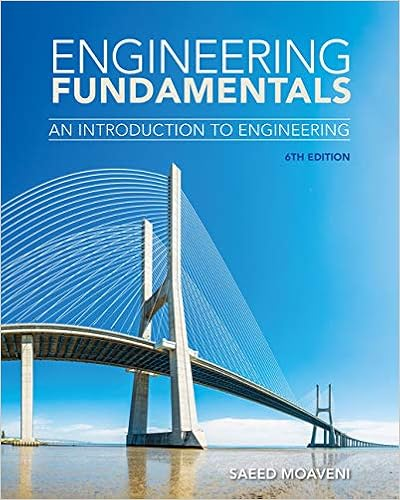Discussion+session5 (1).pptx - ENGR 30 STATICS Discussion...

• 28

This preview shows page 1 - 8 out of 28 pages.

We have textbook solutions for you!
The document you are viewing contains questions related to this textbook.The document you are viewing contains questions related to this textbook.
Chapter 7 / Exercise 3
Engineering Fundamentals: An Introduction to Engineering
MoaveniExpert Verified
ENGR 30 STATICS Discussion Session 5&6 Winter 2018 1
We have textbook solutions for you!
The document you are viewing contains questions related to this textbook.The document you are viewing contains questions related to this textbook.
Chapter 7 / Exercise 3
Engineering Fundamentals: An Introduction to Engineering
MoaveniExpert Verified
Two-force member Requiring that the sum of forces and total moment in any direction be zero leads to the conclusion that F 1 and F 2 must have equal magnitude but opposite sense, and on the same line. Three-force member Since the rigid body is in equilibrium, the sum of the moments of F 1 , F 2 , and F 3 about any axis must be zero. It follows that the moment of F 3 about D must be zero as well and that the line of action of F 3 must pass through D . The lines of action of the three forces must be concurrent or parallel. 7 Fundamental Concepts
Sample Problem A slender rod of length L is attached to collars that can slide freely along the guides shown. Knowing that the rod is in equilibrium, derive an expression for the angle θ in terms of the angle β. W A G B θ β β A B O W A B tan L cos 2 L sin 2 tan GB L sin 2 GO L cos Therefore : tan GO Where : 1 1 1 Fromthe force geometry : GB
Sample Problem Two force member, the force at reactions D and C must pass points DC B x B y
Fundamental Concepts Idealized 3D structural supports 5
Fundamental Concepts Idealized 3D structural supports (for some problem, the couple-moment must be assumed zero to provide statical determinacy, if there are no active forces on that directions) 6
Fundamental Concepts Idealized 3D structural supports 7
•••